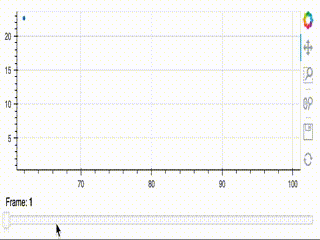# JS callback not working consistently with IndexFilter

Hi!

I’m running into some weird behavior combining JS callbacks and `IndexFilter` that I can’t figure out. Basically if I use `CustomJS` to update the indices of an `IndexFilter` to animate a single point, eventually at a high enough frame number the plot just stops updating. There aren’t any errors in the Chrome Inspector, and the exact frame at which the plot stops updating depends on how fast I drag the slider. It also only seems to trigger if there’s a single element in the filter indices — simply doubling the data with a `pd.concat` before setting it as the source results in a “normal” animation.

Here’s an animated gif of what it looks like:I’ve added the code and the data I’m using to make that plot to a Gist. If you download the data file to the same directory as the script it should work. I’m using Python 3.9.6 and Bokeh 2.3.3.

Is this a bug, or am I doing something wrong? If it’s the former I’d be happy to post an issue on the repo, but I figured I’d ask here first. Thanks!

Offhand this seems like a bug. I’ve gone ahead and simplified the example code even further

``````import pandas as pd
from bokeh.layouts import column
from bokeh.models import ColumnDataSource, CDSView, CustomJS, IndexFilter, Slider
from bokeh.plotting import figure, show

filter = IndexFilter()

plot = figure(height=200, x_range=(60, 105), y_range=(0, 30))
plot.circle(x="x", y="y", source=source,
view=CDSView(source=source, filters=[filter]))

slider = Slider(start=0, end=60, value=0, step=1)

callback = CustomJS(args={"source": source, "filter": filter}, code="""
filter.indices = [cb_obj.value];
source.change.emit();
""")
slider.js_on_change("value", callback)

show(column(plot, slider))
``````

Please post this with the data in a GitHub Issue

In the mean time you might try to see if a `CustomJSFilter` fares any better.

Thanks @Bryan! I liked your simplified version of the code, so that’s what I used in the GH issue (which is here, for posterity’s sake).

1 Like